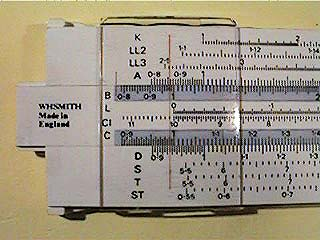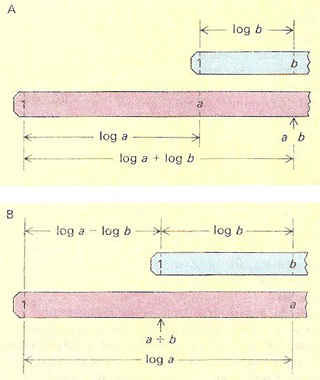A# slide ruleTo multiply using a slide rule [A], for example to find the product of 1.5 and 4, the 1 on the upper scale is lined up with 1.5 on the lower scale. The required product is read off on the lower scale opposite the 4 on the upper – in this example to give 6. The example of division [B] is 6/3. The 3 on the upper scale is lined up with the 6 on the lower scale and the anwer read off opposite the 1 on the upper scale. In this example the required answer is 2.Close-up of part of a slide rule showing the cursor.The slide rule works by the addition and subtraction of the logarithms of numbers. (A) To multiply a by b, the 1 mark on the sliding logarithmic scale (blue) is set opposite the a mark on the fixed logarithmic scale (red). The product a.b is then read off the fixed scale opposite the b mark on the sliding scale. (B) To divide a by b, the b mark on the sliding scale is set opposite the a mark on the fixed scale. The quotient a/b is then read of the fixed scale opposite the 1 mark on the sliding scale. A standard 250mm long slide rule will give answers correct to about 3 significant figures.A cylindrical slide rule can be pictured as a set of long scales wound round the cylinder like a screw thread.

A slide rule is mechanical device based on logarithms which, before the advent of electronic calculators, was used for rapid, though approximate, calculations. Logarithmic scales are engraved on rods that can be slid in relation to each other. and numbers on them added or subtracted as needed – added for multiplication and subtracted for division. Because of the log scale, the numbers become closer together along the slide, just like the frets on a guitar's fingerboard. Unlike an ordinary ruler the scale is geometric rather than arithmetic.

Two scales are calibrated identically so that, on each, the distance from the "1" point to any point on the scale is proportional to the logarithm of the number represented by that point. Since log (a.b) = log a + log b, the multiplication a.b can be performed by setting the "1" point of the scale (1) against a on scale (2), then reading off the number of scale (2) opposite b on scale (1). Division is performed by revering the procedure. A transparent sliding saddle called a cursor can be moved along to line up the graduations and make then easier to read.

The accuracy of a slide rule is limited mainly by its length. A cylindrical slide rule has scales up to a meter long wound round it like a screw thread. Most ordinary slide rules have several different scales for different kinds of calculations: reciprocal (a scale of all the numbers divided into 1), square (numbers multiplied by themselves), square root, and even trigonometrical functions such as sine, cosine, and tangent.